# 12V to 5V Buck Converter Circuit using MC34063

Published  July 20, 2018   4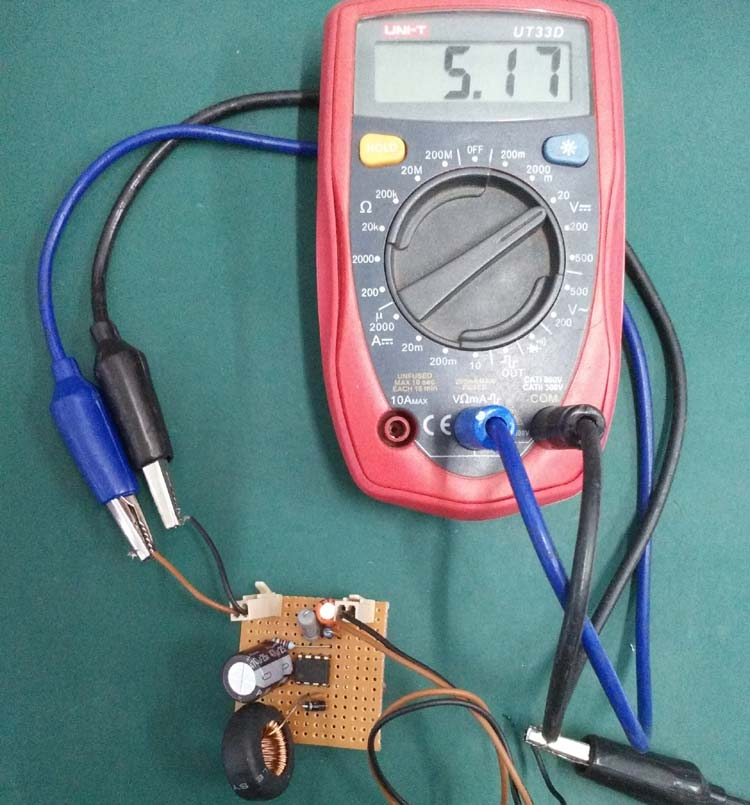In the previous tutorial, we demonstrated detailed design of Boost Converter using MC34063, where a 3.7V to 5V boost converter was designed. Here we see how to convert 12V to 5V. As we know that exact 5V batteries are not always available, and sometimes we need higher voltage and lower voltage at the same time to drive different parts of circuit, so we use higher voltage (12v) source as the main power source and step down this voltage to lower voltage (5v) wherever required. For this purpose, a Buck Converter Circuit is used in many electronics applications which drops the input voltage as per the load requirement.

There are lots of choices available in this segment; as seen in the previous tutorial, MC34063 is one of those most popular switching regulators available in such segment. MC34063 can be configured in three mode, Buck, Boost, and Inverting. We will use the Buck configuration to convert the 12V DC source to 5V DC with 1A output current capability. We have previously built simple Buck Converter circuit using MOSFET; you can also check many more useful power electronics circuits here.

### IC MC34063

MC34063 pinout diagram has been shown in the below image. On the left side the internal circuit of MC34063 is shown, and on the other side the pinout diagram is shown.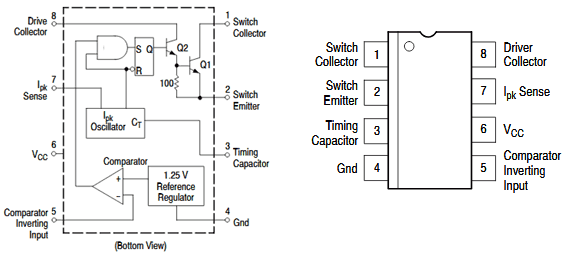MC34063 is a 1.5A Step up or step down or inverting regulator, due to DC voltage conversion property, MC34063 is a DC-DC converter IC.

This IC provides following features in its 8 pin package-

1. Temperature compensated reference
2. Current limit circuit
3. Controlled Duty cycle oscillator with an active high current driver output switch.
4. Accept 3.0V to 40V DC.
5. Can be operated at 100 KHz switching frequency with a 2% tolerance.
6. Very low Standby current

Also, despite these features, it is widely available and it is much cost efficient than other ICs available in such segment.

In the previous tutorial, we designed voltage step-up circuit using MC34063 to boost 3.7V Lithium battery voltage to 5.5V, in this tutorial we will design 12V to 5V Buck converter.

### Calculating the Components’ Values for Boost Converter

If we check the datasheet, we can see the complete formula chart is present to calculate the desired values required as per our requirement. Here is the formula sheet available inside the datasheet, and the step up circuit is also shown.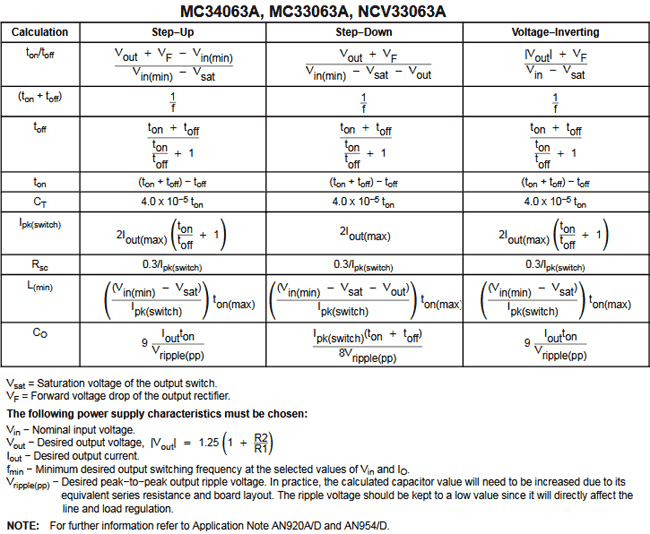Here is the schematic without those components value, which will be used additionally with the MC34063.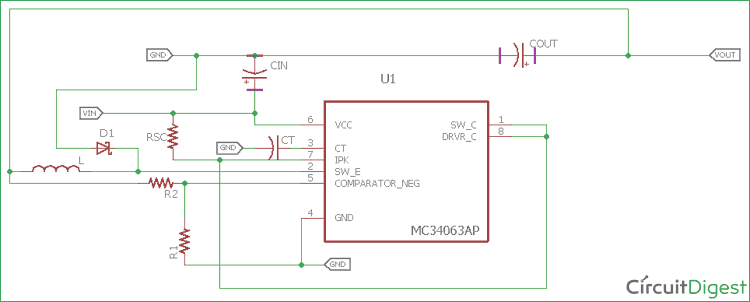We will calculate the values which are required for our design. We can make the calculations from the formulas provided in the datasheet or we can use the excel sheet provided by ON Semiconductor’s website.

Here is the link of the excel sheet.

https://www.onsemi.com/pub/Collateral/MC34063%20DWS.XLS

Steps to calculate those components values-

Step 1:- First, we need to select the Diode. We will choose widely available diode 1N5819. As per the datasheet, at 1A forward current the forward voltage of the diode will be 0.60 V.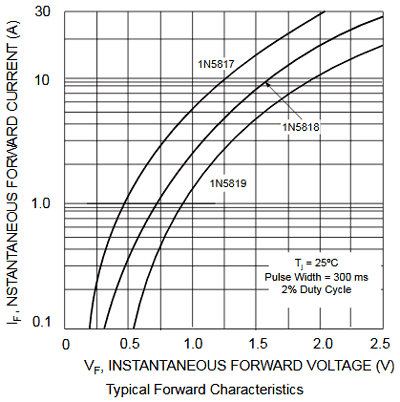Step 2:- We first calculate inductor and switching current as it will be required for further calculation. Our Average Inductor current will be the peak inductor current. So, in our case Inductor current is:

`IL(avg) = 1A`

Step 3:- Now it is time for the ripple current of the inductor. A typical inductor use 20-40% of the Average output current. So, if we choose the inductor ripple current 30%, it will be 1A * 30% = 0.30A

Step 4:- The Switching peak current will be IL(avg) + Iripple/2 = 1 + .30/2 = 1.15A

Step 5:- We will calculate the tON/tOFF using the formula below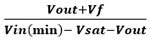For this, our Vout is 5V, and forward voltage of the diode (Vf) is 0.60V. Our minimum input Voltage Vin (min) is 12V and the saturation voltage is 1V (1V in the datasheet). By, putting this all together we get

```(5+0.60) / (12-1-5) = 0.93
So,  tON/tOFF = .93uS```

Step 6:- Now we will calculate the Ton + Toff time, as per the formula Ton + Toff = 1 / f

We will select a lower switching frequency, 40Khz.

`So, Ton + Toff = 1 / 40Khz = 25us`

Step 7:- Now we will calculate the Toff time. As we calculated the Ton + Toff and Ton / Toff previously, the calculation will be easier now,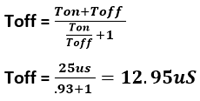Step 8:- Now the next step is to calculate Ton,

`Ton = (Ton + Toff) – Toff = 25us – 12.95us = 12.05us `

Step 9:- We need to choose the timing Capacitor Ct, which will be required to produce the desired frequency.

```Ct = 4.0 x10-5 x Ton = 4.0 x 10-5  x 12.05uS = 482pF
```

Step 10:- Depending on those values we will calculate the Inductor value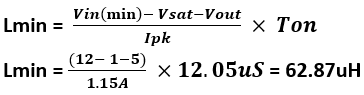Step 11:- For the 1A current, The Rsc value will be 0.3/Ipk. So, for our requirement it will be Rsc = .3/1.15 = .260 Ohms

Step 12:- Let’s calculate the output capacitor values, we can choose a ripple value of 100mV (peak to peak) from the boost output.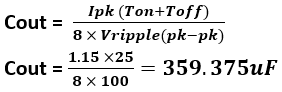We will choose 470uF, 25V. The more capacitor will be used, the more ripple it will reduce.

Step 13:- Last we need to calculate the voltage feedback resistors value. We will choose R1 value 2k, So, the R2 value will be calculated as

```Vout = 1.25 (1 + R2/R1)
5 = 1.25 (1 + R2 / 2K)
R2 = 6.2k```

### Buck Converter Circuit Diagram

So after calculating all the values. Here is the updated schematic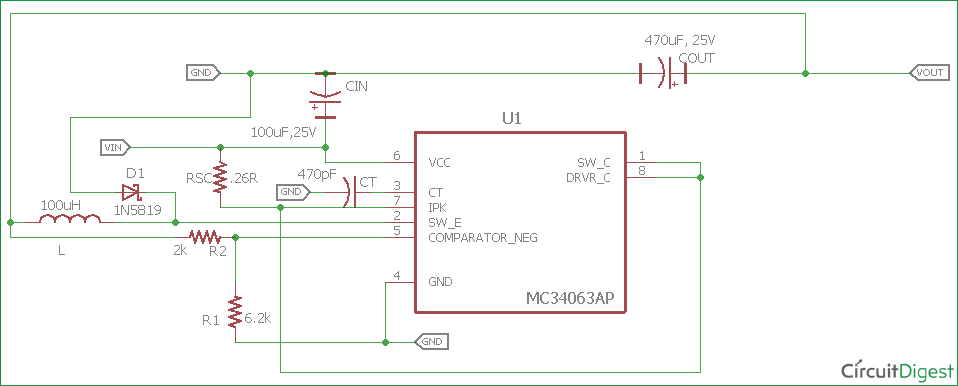### Required components

1. 2 nos relimate connector for input and output
2. 2k resistor- 1 nos
3. 6.2k resistor- 1 nos
4. 1N5819- 1 nos
5. 100uF, 25V and 359.37uF, 25V capacitor (470uF, 25V used, close value selected)- 1 nos each.
6. 62.87uH inductor, 1.5A 1 nos. (100uH 2.5A is used, it was readily available in market)
7. 482pF (470pF used) ceramic disc capacitor- 1 nos
8. 12V Power Supply unit with 1.5A Rating.
9. MC34063 switching regulator ic
10. .26ohms resistor (.3R, 2W used)
11. 1 nos veroboard (dotted or connected vero can be used).
12. Soldering Iron
13. Soldering Flux and Soldering wires.

Note: We have used 100uh inductor as it is available easily with local vendors with the 2.5A current rating. Also we have used .3R resistor instead .26R.

After arranging the components, solder the components on Perf board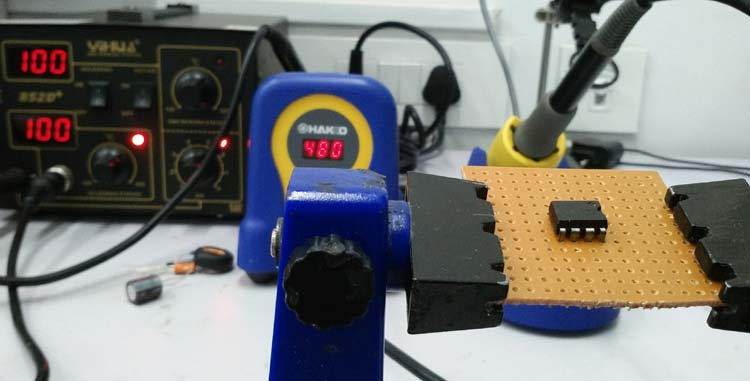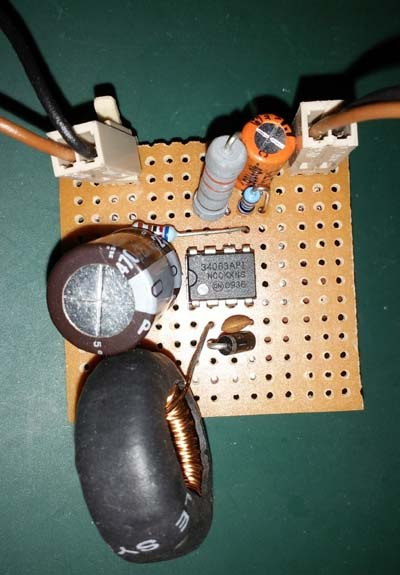### Testing the Buck Converter Circuit

Before testing the circuit we need variable DC loads to draw the current from the DC power supply. In the small electronics lab where we are testing the circuit, test tolerances are much higher and due to that, few measurement accuracies are not up to the mark.

Oscilloscope is properly calibrated but artificial noises, EMI, RF can also change the test result accuracy. Also, the Multimeter have +/-1% tolerances.

Here we will measure the following things

1. Output ripple and voltage at various loads up to 1000mA. Also, test the output voltage at this full load.
2. The efficiency of the circuit.
3. Idle current consumption of the circuit.
4. Short circuit condition of the circuit.
5. Also, what will happen if we overload the output?

Our room temperature is 26 degree Celsius when we tested the circuit.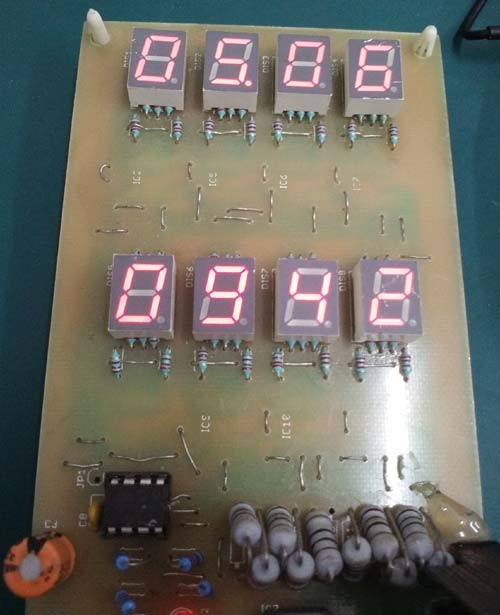In the above image, we can see the DC load. This is a resistive load and as we can see, ten no. of 1 ohm resistors in parallel connection are the actual load, which is connected across a MOS-FET, We will control the MOSFET gate and allow the current to flow through the resistors. Those resistors convert electrical powers to heat. The result consists of 5 % tolerance. Also, these load results include the power draw of the load itself, so when no load is being connected across it and powered using an external power supply, it will show default 70mA of load current. In our case, we will power the load from external bench power supply and test the circuit. The final output will be (Result – 70mA).

Below is our test setup; we have connected the load across the circuit, we measure the output current across the buck regulator as well as the output voltage of it. An oscilloscope is also connected across the buck converter, so we can also check the output voltage. We are providing 12V input from our bench power supply unit.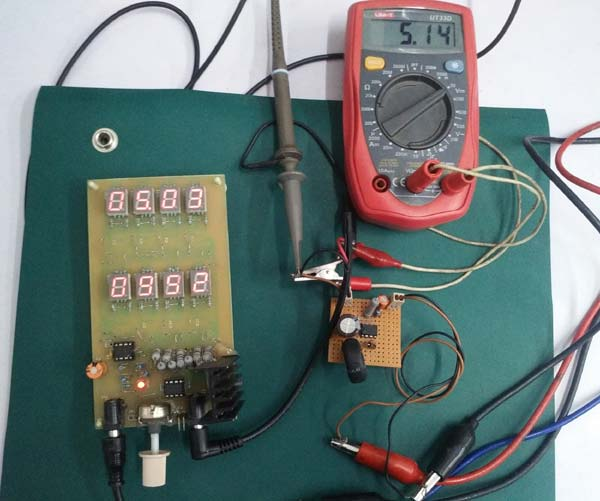We are drawing .88A or 952mA-70mA = 882mA of current from the output. The output voltage is 5.15V.

At this point, if we check the peak to peak ripple in the oscilloscope. We can see the output wave, the ripple is 60mV (pk-pk). Which is good for a 12V to 5V Switching buck converter.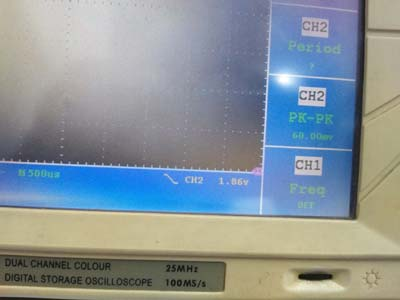The output waveform looks like this: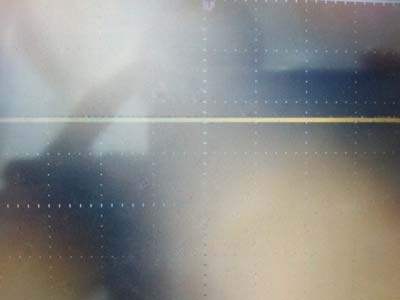Here is the time frame of the output waveform. It is 500mV per division and 500uS time frame.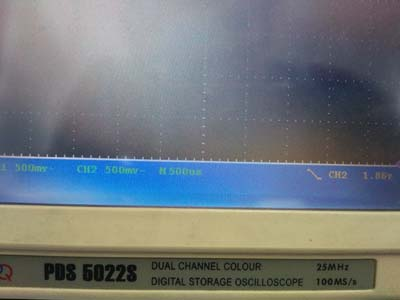Here is the detailed test report

 Time (secs) Load (mA) Voltage (V) Ripple(p-p) (mV) 180 0 5.17 60 180 200 5.16 60 180 400 5.16 60 180 600 5.16 80 180 800 5.15 80 180 982 5.13 80 180 1200 4.33 120

We changed the load and waited for approx 3 minutes, on each step, to check whether the results are stable or not. After 982mA load the voltage dropped significantly. In other cases from 0 loads to the 940 mA, the output voltage dropped was approx .02V, which is quite good stability at full load. Also, after that 982mA load, the output voltage is dropped significantly. We used .3R resistor where .26R was required, due to that, we can draw 982mA of load current. The MC34063 power supply is unable to provide proper stability at full 1A load as we used .3R instead .26R. But 982mA is very close to 1A output. Also, we used resistors with 5% tolerances which are most commonly available at the local market.

We calculated the efficiency at 12V fixed input and by changing the load. Here is the result

 Input Voltage (V) Input Current (A) Input Power (W) Output Voltage (V) Output Current (A) Output Power (W) Efficiency (n) 12.04 0.12 1.4448 5.17 0.2 1.034 71.56699889 12.04 0.23 2.7692 5.16 0.4 2.064 74.53416149 12.04 0.34 4.0936 5.16 0.6 3.096 75.6302521 12.04 0.45 5.418 5.16 0.8 4.128 76.19047619 12.04 0.53 6.3812 5.15 0.98 5.047 79.09170689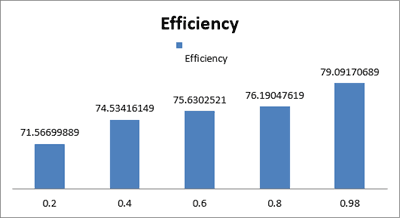As we can see the average efficiency is around 75%, which is a good output at this stage.

Idle current consumption of the circuit is recorded 3.52mA when the load is 0.

Also, we checked for the short-circuit, and we observe Normal in short circuit.

After the maximum output current threshold the output voltages getting significantly lower and after a certain time, it’s getting close to zero.

Improvements can be made in this circuit; we can use low ESR higher value capacitor to reduce the output ripple. Also, proper PCB designing is necessary.

Video

### Buck Converter gives 1.64V only

I have used the same components as mentioned in a document.
also, I gave 12V input voltage using DC 12V power adapter.

But I got only 1.61V in output.
how to get perfect 5v in output?

My circuit looks like this,

Actually, I need to place my 5V operated circuit in every vehicle. So I want to use the vehicle battery as a 12v power supply. That's why I needed 12v to 5v conversion circuit in between.

Any help would be appreciated!!

Thanks!!

### There is a mistake in the…

There is a mistake in the schematic which results in an output voltage of ~1.6V as the other commenter noted.  This is not the only site with the exact same schematic.

The mistake is that the R1 and R2 resistor values are swapped.  The datasheet for the MC34063 says the formula for the output voltage is:

1.25 * (1 + R2 / R1) so as configured that is 1.25 * (1 + 2000 / 6200) = 1.653V.

When swapped, it works correctly:

1.25 * (1 + 6200 / 2000) = 5.13V